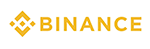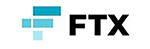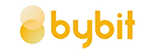메뉴 건너뛰기

### 11. DMI indicator concept and usage

Before explaining the DMI indicator

DMI (Directional Movement Index) - An index that quantifies directional movement as an index.

The DMI is an indicator developed in 1978 by J. Welles Wilder to explain the direction of the price movement of an asset class. This is to check whether the current market trend is valid, and if it is determined that it is valid, you can observe which trend it is moving. It is used to prove validity or to determine strength.

If you search for DMI in the index search, you can use it as follows. The default value is 14.Composition of DMI indicator

The two lines are divided into (+DI) and (-DI). Optionally, the 3rd line, (DX) is also used, which is a line that compares the difference between +DI and -DI.

1. If the (+DI) line is above the (-DI) line, there is upward pressure on the price of a particular asset.

2. If the (-DI) line is above the (+DI) line, there is downward pressure on the price of a particular asset.

3. The intersection of two lines can be used as a trade signal.DMI indicator in the chart

If you look at the Bitcoin chart above, orange = +DI , blue = -DI.

1. When the dominant color line is above the blue line, it indicates that there is upward pressure.

2. When the blue line is above the dominant color line, it indicates that there is downward pressure.

3. These are numbers visualized to observe trends and strengths. It's good to keep in mind when actually trading.

 2021년 해외코인거래소 순위 및 추천거래량 11조(1위) 3.5조 9.5조 레버리지 최대100배 최대100배 최대125배 수수료 - 지정가 : 0.02%           - 시장가 : 0.04%(1위) - 지정가 : -0.025%                - 시장가 : 0.075% - 지정가 : -0.025%                  - 시장가 : 0.075% 거래방법 현물+선물+마진 선물+주식+FOREX 선물 회원가입 회원가입 회원가입 회원가입 할인코드 20% 할인 5% 할인 20% 할인